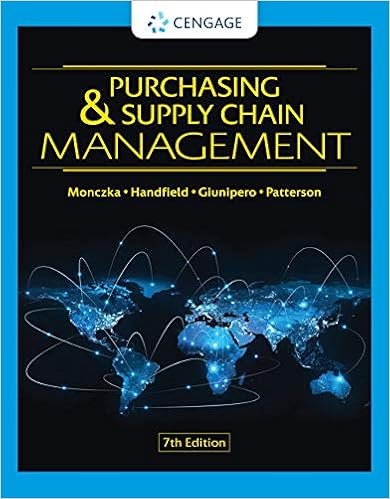# Cost standard input sta b28 dutch fayard expected

• Notes
• Paress
• 9

This preview shows page 7 - 8 out of 9 pages.

##### We have textbook solutions for you!
The document you are viewing contains questions related to this textbook.The document you are viewing contains questions related to this textbook.
Chapter 19 / Exercise 11
Handfield/MonczkaExpert Verified
Expected direct material  cost of one unit of product as determined by the price and input standard. Cost standard =  input standard  x price standardB28:Dutch Fayard:Expected quantity of direct labor used per unit of product. B29:Dutch Fayard:Expected price paid for each hour of direct labor. B30:Dutch Fayard:Expected direct labor cost of one unit of product as determined by the price and input standard. Cost standard =  input standard  x price standardB31:Dutch Fayard:Expected quantity of allocation based used per unit of product. B32:Dutch Fayard:Expected price paid for each unit of allocation base. B33:Dutch Fayard:Expected variable overhead cost of one unit of product as determined by the price and input standard. Cost standard =  input standard  x price standardB34:Dutch Fayard:A flexible budget is a budget developed using the same standards that were used to created the static budget but based on actual production. It is essentially the budget that would have been developed had the actual sales and production output been known.B35:Dutch Fayard:Conceptually, the flexible budget revenues are the revenues we would expect to have given actual sales volume.  Mathmatically, flexible budget revenue equals actual sales volume x  budgeted priceThe difference between static budget revenue and flexible budget revenue is the sales activity variance for revenue.B36:Dutch Fayard:Conceptually, the flexible budget variable costs are the variable costs we expected to incur given actual sales volume.  Mathmatically, flexible budget variable cost equals actual sales volume x  budgted variable cost per unit. This is also equal to standard price x standard quantity allowed for actual sales volumes. The difference between static budget variable costs and flexible budget varible costs is the sales activitiy variance for variable costs. It is also called the sales volume variance for variable costs.B37:Dutch Fayard:Conceptually, the flexible budget fixed costs are the total fixed  costs we expect to incur for the year. They are not generally affected by changes in sales volume, so it should be the same as the static budget fixed costs.B38:Dutch Fayard:Conceptually, the actual revenues are the revenues earned for the year as a result of actual sales volume.  Mathmatically, actual revenue equals actual sales volume x  actual priceThe difference between actual revenues and static budget revenues is the static budget variance.The difference between actual revenues and flexible budget revenues is called the sales price variance.B39:Dutch Fayard:Conceptually, the actual costs are the actual variable costs incurred given actual sales volume.  Mathmatically, actual variable cost equals  actual sales volume x  actual variable cost per unit. B40:Dutch Fayard:Conceptually, actual fixed overhead costs are the total manufacturing related fixed costs incurred during the period. The difference between actual fixed overhead costs and the flexible budget fixed overhead costs (and the static budget fixed overhead costs) is the fixed overhead spending variance.B41:Dutch Fayard:
##### We have textbook solutions for you!
The document you are viewing contains questions related to this textbook.The document you are viewing contains questions related to this textbook.
Chapter 19 / Exercise 11Expert Verified
•••